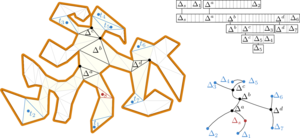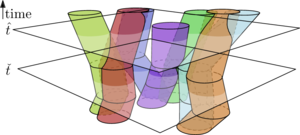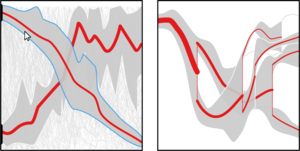# Research Interests

My main research area is in Computational Geometry, an area that focuses on the development of algorithms dealing with geometric data such as points, lines, and circles. My typical approach is to use and develop appropriate theoretical results to solve practical problems from areas such as Geographic Information Science and Visualization. This allows for mathematically sound solutions that have provable properties.

Most of my work is centered around the following four themes.

Movement Analysis (MA)

A large portion of my research has been on algorithms for trajectory and movement analysis. Due to technology such as the Global Positing System (GPS) there is a large amount of trajectory data being collected, and an increasing demand on tools and techniques to analyze such data. I worked on developing such tools and techniques. More recently, I also worked analyzing real time data, using so called kinetic data structures.

Data Structures (DS)

In many algorithms it is of crucial importance that we can store and retrieve the data involved efficiently. Therefore, developing efficient data structures is a key aspect in algorithm design.

Geometric Environments (GE)

In many geometric problems the input data is typically treated as a set of objects, e.g. points, in an empty and infinite space. However, in reality these objects actually “live” in a space that also contains other objects that may interfere with, or otherwise constrain, the problem. Hence, it is important to incorporate such environmental constraints into the original problem.

Information Visualization (IV)
Almost all modern systems capture a large amount of data. To analyze this data and extract useful information out of it, it is important that we can visualize the data appropriately. I worked on the algorithms needed to produce such visualizations.

The problems that I studied usually involved a combination of these themes, and solving them required bringing together knowledge from multiple themes. What follows is a brief overview of some of these problems and how they relate to the themes above. Note that this is only a part of my work. For a full list of my publications see my cv.

## Dynamic Geodesic Nearest Neighbor Searching DSGE

We are given a polygon, and a set of point sites $$S$$ inside the polygon, and we wish to be able to efficiently find the site nearest to an arbitrary query point $$q$$. In addition, we may want to add a new site to $$S$$, or delete an existing one. We designed a fully dynamic data structure that supports updates and such nearest neighbor queries efficiently. This problem originated from a migration problem in ecology, that can now be solved more efficiently.

## Agglomerative Clustering of Growing Squares MADSIV

Consider the process of zooming out on a map. During this process the labels with e.g. place names, may start to overlap. We have to detect this, and decide which labels to remove. We model this problem as a dynamic set of disjoint squares, each square growing in size as a function of time. We maintain the squares in a dynamic data structure so that we can efficiently detect the first time at which two squares start to intersect, and remove and insert squares.

## IO Efficient Shortest Path Problems DSGEThe input polygon, its representation on disk, and its structure.

Technological advances allow us to measure data in the real world more easily and more precisely. This leads to an ever increasing amount of data with which algorithms have to compute. In the traditional model of computation we analyze algorithms by counting the number of basic CPU operations that an algorithm performs. However, if the data can no longer fit in the main memory of a computer then the time it takes to transfer data to and from the disk actually determines the running time of the algorithm. Therefore, we need to analyze algorithms in the IO or the cache oblivious models, which count the number of such memory transfers.

We considered several shortest path problems in the IO model, and developed new algorithms that achieve much better IO efficiency compared to traditional algorithms.

When setting up a communication system it maybe desirable to place a minimum number of transmitters, each consecutive pair mutually visible, to support sending messages from a source to a destination. We showed that several such minimum-link path problems are NP-hard, thereby solving a long outstanding open problem.

## Trajectory Grouping Structure under Geodesic Distance MAGEThe structure of moving entities joining and splitting over time.

Ecologists wish to analyze movement patterns such as flocks and groups in the trajectory data of animals. A group consists of sufficiently many entities (animals) that move together during a sufficiently long time interval. In addition to the groups themselves, we want to find the relations between groups. For example, a large group was born when two smaller groups merged. See these videos for some examples.

We designed efficient algorithms that can find all groups in a collection of trajectory data. Moreover, we can do so even when the entities move in a constrained geometric environment. This greatly influences when entities should be considered together.

## Grouping Time-varying Data for Interactive Exploration MADSIV

The definition of a group in the problem above contains some threshold $$\eps$$ on the allowed distance between entities. In many cases, ecologists do not actually know the right value of $$\eps$$ in advance, and hence they may wish to use the above algorithm to find the right value $$\eps$$ by interactively exploring the grouping data. We developed efficient data structures to compute and store the groups that allows the user to do so.

## Multi-Granular Trend Detection for Time-Series Analysis MAIVTwo examples of our trend detection visualization tool.

We are given a large amount of time-series data, for example weather forecasts, and we wish to visualize “trends” in the data. That is, we want to detect and visualize whether there are several time series that behave similarly over an extended period of time. We developed algorithms to compute such trends, and implemented a tool that allows users to visualize them.